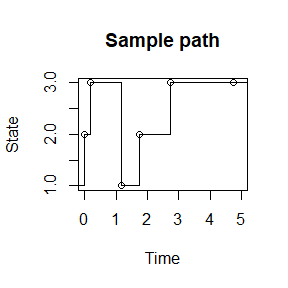# Simulating sample paths for endpoint-conditioned continuous time Markov chains via the ECctmc package

#### 2018-05-01

The ECctmc package is a lightweight C++ implementation of the modified rejection sampling and uniformization algorithms detailed in Hobolth and Stone (2009). These algorithms allow users to efficiently simulate sample paths for continuous time Markov chains (CTMC) with discrete state spaces conditional on the state of the chain at the endpoints of the sampling interval. This implementation assumes that state sojourn times are exponentially distributed, and that the process is time-homogeneous. In this vignette we will briefly outline the two algorithms and demonstrate how to call the sampling functions to obtain a collection of paths. This vignette is not intended to be a completely describe the mathematical details of the algorithms or their relative efficiency. For details on the modified rejection sampling and uniformization algorithms, we suggest the Hobolth and Stone (2009) paper, which is really quite excellent.

## Notation

We are interested in sample paths for a finite state CTMC, $$X(t)$$, over the time interval [0, T], conditional on $$X(0) = a$$ and $$X(T) = b$$. We suppose that the chain has rate matrix $$Q$$, where $$Q_{i,j}$$ denotes the instantaneous rate of change from state $$i$$ to state $$j$$, and $$Q_i = Q_{i,i} = -\sum_{j\neq i} Q_{i,j} > 0$$ is the rate of exit out of state $$i$$. We will use $$\tau$$ to denote waiting times.

## Forward sampling - Gillespie’s direct algorithm

1. Sample $$\tau\sim exp(Q_a)$$. If $$\tau>T$$, then $$X(t) = a \forall t\in[0, T]$$.
2. If $$\tau < T$$, sample the next state, $$j$$ from the discrete probability distribution with masses given by $$\frac{Q_{a,j}}{Q_a}$$, for $$j\neq a$$. Repeat step 1, starting in $$X(\tau)=j$$.

Simple rejection sampling retains only the paths where $$X(T)=b$$.

## Modified rejection sampling

If $$a=b$$,

1. Draw a path using the forward sampling algorithm, outlined above.
2. Accept the path if $$X(T)=a$$. Otherwise, repeat step 1.

If $$a\neq b$$,

1. Sample the first time of state transition using the inverse-CDF method. i.e. For $$u\sim Unif(0,1)$$, the distribution of $$\tau$$, given that at least one state transition occurs, may be sampled from by transforming $$u$$ by $F^{-1}(u) = -log(1 - u(1 - \exp(-Q_a T)))/Q_a$
2. Sample the next state, $$j$$ from the discrete probability distribution with masses given by $$\frac{Q_{a,j}}{Q_a}$$, for $$j\neq a$$.
3. Simulate the rest of the path in the interval $$[\tau, T]$$ using the forward sampling algorithm, starting at $$X(\tau) = j$$.
4. Accept the path if $$X(T)=b$$. Otherwise, return to step 1.

Modified rejection sampling improves upon simple rejection sampling by avoiding sampling constant paths. It will be efficient when the time interval is small and the path is non-constant. However, when transitions into the final state are unlikely, many paths will be rejected.

## Uniformization

We begin by defining an auxilliary stochastic process, $$Y(t)$$, for which we construct a transition matrix $R = I + Q/\mu$ where $$\mu = \max_i Q_i$$. Let $$R^n_{i,j}$$ denote the $$i,j$$ element of the $$n^{th}$$ power of $$R$$. Let $$P_(T)=e^{QT}$$ be the discrete transition probability matrix of $$X(t)$$ over the interval, and $$P_{a,b}(T)$$ be it’s $$a,b$$ element. We proceed as follows:

1. Let $$u\sim Unif(0,1)$$. Sample the number of jumps in $$[0,T]$$ conditional on $$X(0)=a$$ and $$X(T)=b$$ according to the discrete distribution with masses given by $\Pr(N=n | X(0)=a, X(T)=b) = e^{\mu T} \frac{(\mu T)^n}{n!}R^n_{a,b}/P_{a,b}(T)$ by taking $$n$$ to be the first time that the cumulative sum of the probability masses above exceeds $$u$$. Save the matrices $$R^n$$ in these cumulative sums.
2. If $$n=0,\ X(t)=a\ \forall t\in [0,T]$$.
3. If $$n=1$$ and $$a=b,\ X(t)=a\ \forall t\in [0,T]$$.
4. If $$n=1$$ and $$a\neq b$$, draw $$\tau\sim Unif(0,T)$$. Then $$X(t)=a,\ 0\leq t < \tau$$, and $$X(t)=b,\ \tau \leq t \leq T$$.
5. If $$n\geq 2$$, sample the times of state transition, $$\tau_1,\dots,\tau_n$$, uniformly and independently within the interval $$[0,T]$$ and order. Then, sample the sequence of states $$X(\tau_i),\ i=1,\dots,n-1,$$ from the discrete distribution with masses given by $\Pr(X(\tau_i) =x_i | X(\tau_{i-1}) = x_{i-1}, X(T)=b)) = \frac{R_{x_{i-1},x_i} (R^{n-i})_{x_i,b}}{(R^{n-i+1})_{x_{i-1},b}}$

## Simulating sample paths

We may simulate sample paths by calling the sample_path() function, which returns either a matrix containing the CTMC path, or a list of matrices each containing a path. This function has only a few argments that must be specified. There are:

• a,b: states at the interval endpoints, each of which is in 1,…,# states.
• t0,t1: times of the interval endpoints.
• Q: CTMC rate matrix.

The default number of paths is 1, although the user can request any number of paths to be returned in a list. We can optionally specify a method, either “mr”, or “unif”, though the default is modified rejection sampling. If uniformization is specified, the user can optionally provide a vector of eigen values, the matrix of corresponding eigen vectors, and the inverse eigen vector matrix. This may help speed up computations if the eigen decomposition has been computed beforehand. When multiple paths are requested, the eigen decomposition is computed once and cached for re-use, so there is no need to supply a pre-computed eigen decomposition.

set.seed(183427)
require(ECctmc)
## Loading required package: ECctmc
# rates
r1 <- 1 # 1->2
r2 <- 0.75 # 2->3
r3 <- 0.5 # 3->1
r4 <- 0.5 # 3-> 2
Q <- matrix(c(-r1, r1, 0, 0, -r2, r2, r3, r4, -(r3+r4)), nrow = 3, byrow = TRUE)

# sample path
path <- sample_path(a=1, b=3, t0=0, t1=5, Q=Q)
path
##           time state
## [1,] 0.0000000     1
## [2,] 0.1631161     2
## [3,] 1.1726034     3
## [4,] 1.7334418     1
## [5,] 2.7399563     2
## [6,] 4.7602188     3
## [7,] 5.0000000     3
plot(stepfun(x=path[1:(nrow(path)-1),"time"], y = path[,"state"]), xlim = c(0,5), xlab = "Time", ylab = "State", main = "Sample path")